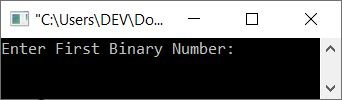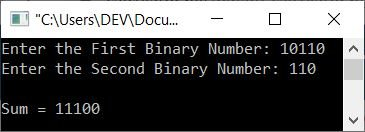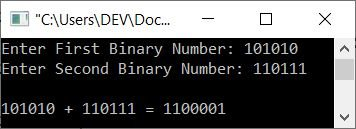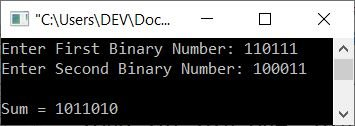# C++ Program to Add Two Binary Numbers

In this article, you'll learn and get code for the addition of two binary numbers entered by the user at run-time. Here I've created programs for binary number addition in the following ways:

Note: If you don't know the basic rules of binary number addition, you can refer to its separate tutorial.

## In C++, add binary numbers using the string data type

The question is: write a program in C++ that receives two binary numbers as input and finds and prints their addition. The program given below uses string data types to do the task. The size() function returns the length of a string.

```#include<iostream>
using namespace std;

int main()
{
int len, carry, re, i;
cout<<"Enter First Binary Number: ";
cin>>bin1;
cout<<"Enter Second Binary Number: ";
cin>>bin2;
len = bin1.size();
carry = 0;
for(i=(len-1); i>-1; i--)
{
re = carry;
if(bin1[i] == '1')
re = re+1;
else
re = re+0;
if(bin2[i] == '1')
re = re+1;
else
re = re+0;
if(re%2==1)
else
if(re<2)
carry = 0;
else
carry = 1;
}
if(carry!=0)
return 0;
}```

Here is the initial output produced by the above C++ program:Now enter 11101 as the first binary number and 11111 as the second. Here is its sample run with these user inputs:The dry run of the above program with the same user input, that is, 11101 and 11111 as two binary numbers, goes like this:

• When the user enters these two binary inputs, it gets stored in bin1 and bin2 variables respectively. That is of string type. Here is the character-by-character storage of bin1 = "11101" and bin2 = "11111":
• bin1 = '1'
• bin1 = '1'
• bin1 = '1'
• bin1 = '0'
• bin1 = '1'
• In a similar way, all characters get stored at their respective indexes for second input in bin2.
• Now, using the statement
`len = bin1.size();`
The length of the first binary number gets initialized to len. Therefore len=5, because there are 5 characters available in bin1
• The empty string, that is "" and an empty integer, that is 0 get initialized to two variables, addRes and carry, respectively.
• Now the execution of the for loop begins, starting with i's value from len-1, 5-1, or 4.
• Since the condition i>-1 or 4>-1 evaluates to be true, the program flow goes inside the loop and evaluates all the statement(s) available inside its body.
• That is, the first statement
`re = carry;`
gets executed. Therefore re=0
• Now that the condition (of first if), bin1[i] == "1" or bin == "1" or "1" == "1" evaluates to True, re+1, 0+1, or 1 is initialized to re.
• Again, the condition (of the second if), bin2[i] == "1" or bin2 == "1" or "1" == "1" evaluates to True, so re+1, 1+1, or 2 is initialized to "re."
• Again, the condition (of the third if), re%2==1 or 2%2==1, evaluates to false, so program flow proceeds to its inverse, else, and '0' + addRes or '0' + "" or '0' is initialized to addRes.
• Again the condition (of fourth and last if), re<2 or 2<2 evaluates to be False, therefore program flow goes to else's body and 1 gets initialized to carry. So carry = 1
• Since all the statements get executed, the program flow goes to the increment part of the loop, and the value of i gets decremented. Now i=3
• Then the condition i>-1 or 3>-1 again evaluates to be true, therefore the program flow again goes inside the loop. This process continues, until the condition is evaluated as false.
• After exiting from the loop, or when the condition (i>-1) evaluates to be false, "1" gets added before the addRes variable, but only if carry does not equal 0.
• In this way, the addition of two binary numbers gets calculated.

Note: The above program has a limitation. The limitation is that if the length of two entered binary inputs is not the same or equal, the program doesn't work correctly. Therefore, here is another program created that works in all conditions, whether the length of binary number inputs is equal or not.

```#include<iostream>
using namespace std;

int main()
{
string str1, str2, sum="";
int len1, len2, i, j, ds=0;
cout<<"Enter the First Binary Number: ";
cin>>str1;
cout<<"Enter the Second Binary Number: ";
cin>>str2;
len1 = str1.size();
len2 = str2.size();
i = len1 - 1;
j = len2 - 1;
while(i>=0 || j>=0 || ds==1)
{
ds = ds + ((i >= 0) ? str1[i] - '0' : 0);
ds = ds + ((j >= 0) ? str2[j] - '0' : 0);
sum = char(ds % 2 + '0') + sum;
ds = ds/2;
i--;
j--;
}
cout<<"\nSum = "<<sum;
return 0;
}```

Here is its sample run with user inputs 10110 and 110 as two binary numbers:## In C++, addition of a binary number using the int data type

This is the program you need, I think. Because this program does not use any string type to do the task. This program is created with all its variables as int types. However, the output of both programs is identical.

```#include<iostream>
using namespace std;

int main()
{
long int bin_one, bin_two, t1, t2;
int i=0, rem=0, sum;
cout<<"Enter First Binary Number: ";
cin>>bin_one;
cout<<"Enter Second Binary Number: ";
cin>>bin_two;

t1 = bin_one;
t2 = bin_two;

while(bin_one !=0 || bin_two !=0)
{
sum[i++] = (bin_one % 10 + bin_two % 10 + rem) % 2;
rem = (bin_one % 10 + bin_two % 10 + rem) / 2;
bin_one = bin_one/10;
bin_two = bin_two/10;
}

if(rem!=0)
sum[i++] = rem;

i--;
cout<<endl<<t1<<" + "<<t2<<" = ";

while(i>=0)
cout<<sum[i--];

return 0;
}```

Here is its sample run with user input: 101010 as the first and 110111 as the second binary number:## In C++, add binary numbers using a user-defined function

Here is another program that does the same task as the previous two programs but uses a user-defined function named addBinNums() that takes two arguments. One for the first binary number entered by the user at run-time, and the other for the second binary number.

```#include<iostream>
using namespace std;

int i=0, sum;
int main()
{
long int bin_one, bin_two;
cout<<"Enter First Binary Number: ";
cin>>bin_one;
cout<<"Enter Second Binary Number: ";
cin>>bin_two;

cout<<endl<<"Sum = ";
while(i>=0)
cout<<sum[i--];

return 0;
}
{
int r=0;
while(b1 !=0 || b2 !=0)
{
sum[i++] = (b1 % 10 + b2 % 10 + r) % 2;
r = (b1 % 10 + b2 % 10 + r) / 2;
b1 = b1/10;
b2 = b2/10;
}
if(r!=0)
sum[i++] = r;
i--;
}```

Here is its sample run with user input, 110111 and 100011 as first and second binary numbers:In the above program, the statement int i=0, sum; is declared globally, which is outside of both the main() and addBinNums() functions. So that we can use these two variables throughout the program along with their updated values.

C++ Quiz

« Previous Program Next Program »